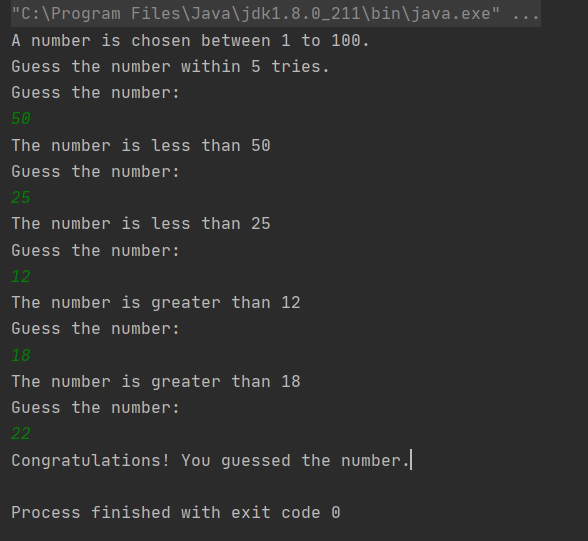# Number guessing game in Java

The task is to write a Java program in which a user will get K trials to guess a randomly generated number. Below are the rules of the game:

• If the guessed number is bigger than the actual number, the program will respond with the message that the guessed number is higher than the actual number.
• If the guessed number is smaller than the actual number, the program will respond with the message that the guessed number is lower than the actual number.
• If the guessed number is equal to the actual number or if the K trials are exhausted, the program will end with a suitable message.

Approach: Below are the steps:

• The approach is to generate a random number using Math.random() method in Java.
• Now using a loop, take K input from the user and for each input print whether the number is smaller or larger than the actual number.
• If within K trials the user guessed the number correctly, print that the user won.
• Else print that he was not able to guess and then print the actual number.

Below is the implementation of the above approach:

## Java

 `// Java program for the above approach ` `import` `java.util.Scanner; ` ` `  `public` `class` `GFG { ` ` `  `    ``// Function that implements the ` `    ``// number guessing game ` `    ``public` `static` `void` `    ``guessingNumberGame() ` `    ``{ ` `        ``// Scanner Class ` `        ``Scanner sc = ``new` `Scanner(System.in); ` ` `  `        ``// Generate the numbers ` `        ``int` `number = ``1` `+ (``int``)(``100` `                               ``* Math.random()); ` ` `  `        ``// Given K trials ` `        ``int` `K = ``5``; ` ` `  `        ``int` `i, guess; ` ` `  `        ``System.out.println( ` `            ``"A number is chosen"` `            ``+ ``" between 1 to 100."` `            ``+ ``"Guess the number"` `            ``+ ``" within 5 trials."``); ` ` `  `        ``// Interate over K Trials ` `        ``for` `(i = ``0``; i < K; i++) { ` ` `  `            ``System.out.println( ` `                ``"Guess the number:"``); ` ` `  `            ``// Take input for guessing ` `            ``guess = sc.nextInt(); ` ` `  `            ``// If the number is guessed ` `            ``if` `(number == guess) { ` `                ``System.out.println( ` `                    ``"Congratulations!"` `                    ``+ ``" You guessed the number."``); ` `                ``break``; ` `            ``} ` `            ``else` `if` `(number > guess ` `                     ``&& i != K - ``1``) { ` `                ``System.out.println( ` `                    ``"The number is "` `                    ``+ ``"greater than "` `+ guess); ` `            ``} ` `            ``else` `if` `(number < guess ` `                     ``&& i != K - ``1``) { ` `                ``System.out.println( ` `                    ``"The number is"` `                    ``+ ``" less than "` `+ guess); ` `            ``} ` `        ``} ` ` `  `        ``if` `(i == K) { ` `            ``System.out.println( ` `                ``"You have exhausted"` `                ``+ ``" K trials."``); ` ` `  `            ``System.out.println( ` `                ``"The number was "` `+ number); ` `        ``} ` `    ``} ` ` `  `    ``// Driver Code ` `    ``public` `static` `void` `    ``main(String arg[]) ` `    ``{ ` ` `  `        ``// Function Call ` `        ``guessingNumberGame(); ` `    ``} ` `} `

Output:
Below is the output of the above program:Attention reader! Don’t stop learning now. Get hold of all the important DSA concepts with the DSA Self Paced Course at a student-friendly price and become industry ready.

My Personal Notes arrow_drop_upIf you like GeeksforGeeks and would like to contribute, you can also write an article using contribute.geeksforgeeks.org or mail your article to contribute@geeksforgeeks.org. See your article appearing on the GeeksforGeeks main page and help other Geeks.

Please Improve this article if you find anything incorrect by clicking on the "Improve Article" button below.

Article Tags :
Practice Tags :

Be the First to upvote.

Please write to us at contribute@geeksforgeeks.org to report any issue with the above content.